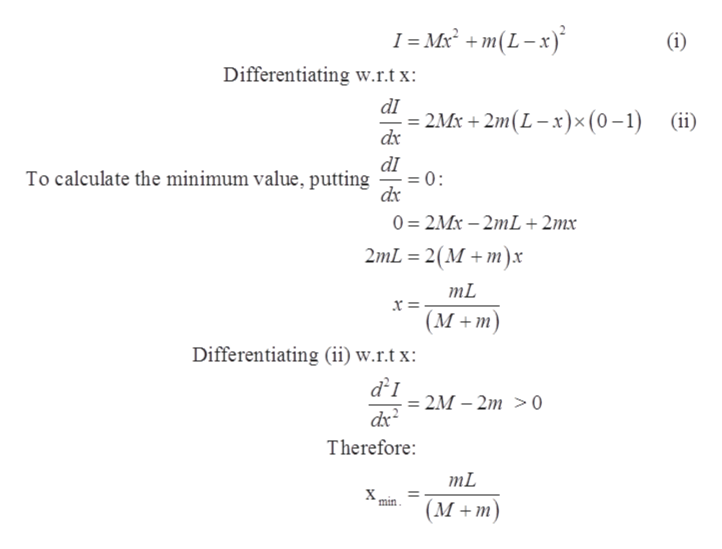# Two balls with masses M and m are connected by a rigidrod of length L and negligible mass as shown in FigureP10.28. For an axis perpendicular to the rod, (a) show thatthe system has the minimum moment of inertia when theaxis passes through the center of mass. (b) Show that this R moment of inertia is I = uL2, where u=mM/(m 1 M).

Question
193 views

Two balls with masses M and m are connected by a rigid
rod of length L and negligible mass as shown in Figure
P10.28. For an axis perpendicular to the rod, (a) show that
the system has the minimum moment of inertia when the
axis passes through the center of mass. (b) Show that this R moment of inertia is I = uL2, where u=mM/(m 1 M).

check_circle

star
star
star
star
star
1 Rating
Step 1

Given:

Masses of the balls are M and m respectively.

Length of the rod, = L

We know that moment of inertia around the axis:

Step 2

(a)

Writing the moment of inertia from the fig.help_outlineImage TranscriptioncloseI Mx +m(L-x) (i) Differentiating w.r.tx dI - 2Mr + 2m(L- х)x (0-1) dx (i) dl To calculate the minimum value, putting 0: dx 0 2Mx 2mL+2mx 2mL= 2(M +m)x mL x = (M +m Differentiating (ii) w.r.t x 3D 2М - 2т > 0 dx2 Therefore: mL х. min (M +m) fullscreen
Step 3

Which is also the center of...

### Want to see the full answer?

See Solution

#### Want to see this answer and more?

Solutions are written by subject experts who are available 24/7. Questions are typically answered within 1 hour.*

See Solution
*Response times may vary by subject and question.
Tagged in

### Other